+

# Coefficient of Determination/r^2

##### Rating:
(8)
• (3)
• (2)
• (0)
• (0)
• (3)
Author: Sophia Tutorial
##### Description:

Relate the correlation coefficient to the coefficient of determination in a given situation.

(more)
Tutorial
what's covered
This tutorial will explain the coefficient of determination. This is also called r squared, the square of the correlation coefficient. Our discussion breaks down as follows:

1. The Coefficient of Determination
2. Finding r From r-squared

## 1. The Coefficient of Determination

The correlation coefficient, r, gives a general measure of strength and direction of a linear relationship. There is also the coefficient of determination, or r squared, which provides a very specific measurement. It provides the percent of the variation in the y-direction that can be explained by the linear relationship with the x variable. This can be a little confusing to understand.

EXAMPLE

Even though it is on the y-axis, the graph here is a dot plot of the seafood prices in 1980. This is going to be your y variable, but it's not very well contextualized. You would still wonder why the point all the way up at 400, which represents sea scallops, are so expensive. What would cause that to be so high, while other prices are so low?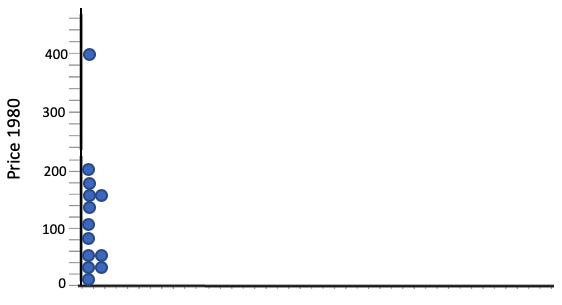What you can do is add a variable to understand why the 1980 price of sea scallops was so high, while some of the other prices were so low. Look at it with the new variable of 1970 prices to explain why some of these are high or low or in the middle.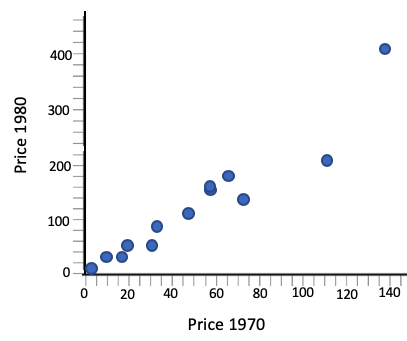The low prices were low in 1970, and the high prices were high in 1970. Looking at this separated of its previous context doesn't really help to explain why certain prices are high or low. Looking at it with the full context of previous knowledge and its associations helps to explain why that specific point is high up, and why some of the other points are low. It's high up because it's strongly linearly associated.
The value of the coefficient of determination, or r2, in this particular example is 0.935. This means that 93.5% of the variation in 1980 prices can be explained by a linear association with 1970 prices.

think about it
You might be wondering what happened to the other 6.5% of the variation. How is that explained? The reason that it's not 100% of the variation is that these points don't all lie perfectly on a line. If they did, all the reasoning behind the 1980 price would be explained by the 1970 price. But, they don't lie exactly on a line.

Some points fall conspicuously a little bit below what you would imagine the line to look like. The remaining 6.5% of variation has to be explained by something else. Perhaps some species of fish were over-fished, and that raised prices. Or perhaps people's tastes changed, and the demand for a particular fish fell, and that lowered the price.
term to know

Coefficient of Determination (r^2)
A value that explains the percent of variation in the response variable that can be explained by a linear association with the explanatory variable. It is the square of the correlation coefficient.

## 2. Finding r From r-squared

Ultimately, r squared is always a positive number, and it does help to measure the strength of the linear association. It measures something very, very specific. It doesn't indicate the direction; it only can indicate the strength.

We can also use the coefficient of determination, r2 to find the correlation coefficient, r.

step by step
Step 1: Take the square root of r2. If only r-squared is given, what you have to do is take the square root to obtain the correlation coefficient, r.
Step 2: Look at the graph to determine sign. You also have to look at the graph to find the association--either positive or negative--to determine the sign of the correlation coefficient.

EXAMPLE

Look at each of the following examples and find the correlation coefficient, r, from r-squared.

Coefficient of Determination (r2) Graph Correlation Coefficient (r)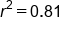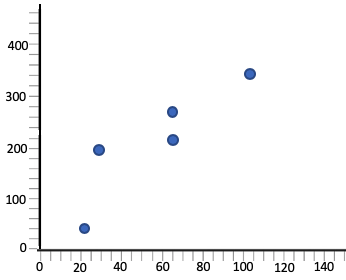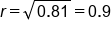Graph shows a positive association, so the correlation coefficient stays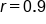.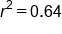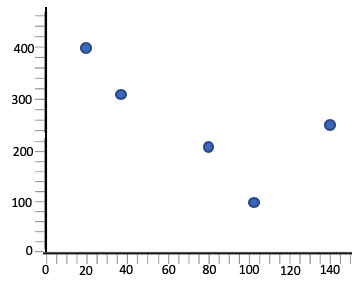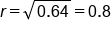Graph shows a negative association, so the correlation coefficient is actually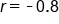.

summary
The coefficient of determination allows us to understand the percent of variation in the vertical direction that can be explained by the linear association that the two variables have. If you solve for r, from r squared, you need to not only take the square root but also look at the scatterplot to determine the sign, because r squared can't be negative but r can.

Good luck!

Source: Adapted from Sophia tutorial by Jonathan Osters.

Terms to Know
Coefficient of Determination (r2)

A value that explains the percent of variation in the response variable that can be explained by a linear association with the explanatory variable. It is the square of the correlation coefficient.

Rating Header